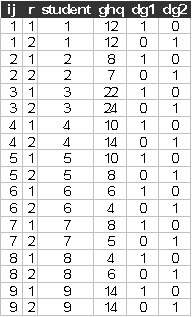Exercise L1, linear model

Dunn (1992) reported data for the 12-item version of Goldberg’s (1972) General Health Questionnaire for psychological distress. The questionnaire was completed by 12 students on 2 dates, 3 days apart. The data are repeated in the table below, the same data were used by Rabe-Hesketh and Skrondal (2005, exercise 1.2).

Data description

Number of observations (rows):12

Number of variables (columns): 3

Variables:

ij= student identifier

r = response occasion 1, 2

student =student identifier {1,2,…,12}

ghq = psychological distress score at occasion

dg1=1, if the response occasion is 1, 0 otherwise

dg2=1, if the response occasion is 2, 0 otherwise

The data (ghq2.dat) look like:Start Sabre and specify transcript file:

out ghq.log

data ij r student ghq dg1 dg2

read ghq2.dat

Suggested exercise:

(1) Estimate the linear model in sabre on ghq, with just a constant, and no random effects.

(2) Estimate the linear model, allowing for the student random effect, use mass 64 and starting value sigma 1. Are the student random effects significant? What does the significance mean? What impact do the student random effects have on the model?

(3) Re-estimate the linear model allowing for both student random effects and dg2. How do the results change (compared to part 2)?

References

Dunn, G., (1992), Design and analysis of reliability studies, Statistical Methods in Medical Research, 1, 123-157

Rabe-Hesketh, S., and Skrondal, A., (2005), Multilevel and Longitudinal Modelling using Stata, Stata Press, Stata Corp, College Station, Texas